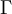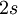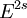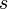2022: SklogWiki celebrates 15 years on-line

# Phase space

Phase space is the name given to a coordinate-momentum space. It is the means by which a mechanical problem can be converted in to a geometrical problem. Phase space, sometimes written as$\Gamma$-space, is an Euclidean space in$2s$ dimensions (i.e.$E^{2s}$), where$s$ is the number of degrees of freedom. Thus a description of a system in terms of positions and velocities now becomes a point in phase space (known as a phase point). Changes in the system now trace out a trajectory in phase space (known as a phase trajectory). Two different phase trajectories cannot pass through the same phase point. One important property of phase space is that, for a long period of time, the phase-trajectory will spend an equal amount of time in equal volume elements.